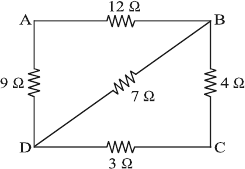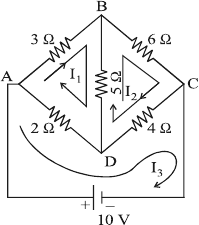MORE IN Basic Electrical Engineering (BEE)
SPPU First Year Engineering (Semester 1)
Basic Electrical Engineering (BEE)
December 2015
Total marks: --
Total time: --
INSTRUCTIONS
(1) Assume appropriate data and state your reasons
(2) Marks are given to the right of every question
(3) Draw neat diagrams wherever necessary

Solve any one question from Q1 and Q2
1 (a) Obtain the equation for coefficient of coupling between two coils placed on common magnetic field.
6 M
1 (b) A water pump lifts 50 m3 of water per hour to a height of 20 m. The overall efficiency of water pump set is 0.60. Find monthly bill, when the pump is used 4 hours a day for a month of 30 days, at the rate of Rs. 5/kWh.
6 M

2 (a) Explain the effect of temperature on resistance of:
(i) metal conductors
(ii) insulators
(iii) alloys.
6 M
2 (b) A steel ring of mean diameter of 50 cm is wound with 500 turns on it. A flux density of 1 Tesla is produced in the ring by mmf of 4000 AT/m. Calculate magnetising current. Also find the current when an air gap of 1 mm is cut in it, keeping B = 1 Tesla in the ring.
6 M

Solve any one question from Q3 and Q4
3 (a) Obtain the expression for composite capacitor having 3-dielectric materials.
7 M
3 (b) A 50 Hz sinusoidal voltage has rms value of 200 V. At t = 0, the instantaneous value is positive and half of its maximum value. Write down the expression for voltage and sketch the wave form.
6 M

4 (a) Derive an equation for average value of sinusoidal current in terms of its peak value.
6 M
4 (b) A 40 kVA, 2200/220 V, 50 Hz, 1-ph transformer has an iron loss of 250 W. The resistances of low and high voltage windings are 0.005 Ω and 0.5 Ω respectively. Calculate efficiency at full load and load p.f. = 0.8 lagging.
7 M

Solve any one question from Q5 and Q6
5 (a) Obtain the expression for power, if x = vm sin out is applied to R-L series circuit.
6 M
5 (b) Three identical impedances each of 6 + j8 Ω are connected in star across 3-ph, 400 V, 50 Hz a.c. supply. Calculate:
(i) line current
(ii) active power
(iii) reactive power.
7 M

6 (a) What is series resonance? Obtain the equation for resonant frequency.
6 M
6 (b) Two circuits having impedances Z1 = 8 + j6 Ω and Z2 = 5 +j10 Ω are connected in parallel across 200 V, 50 Hz, 1-ph a.c. supply. Calculate:
(i) current drawn by each circuit
(ii) total current
(iii) p.f. of whole circuit.
7 M

Solve any one question from Q7 and Q8
7 (a) Using simple series-parallel combination, find RBC for Fig 1.6 M
7 (b) Obtain the equations to convert A-connected resistances into equivalent star circuit.
6 M

8 (a) State the Thevenin's theorem and explain the steps to find Rth, Vth and Ith.
6 M
8 (b) Using Mesh/Loop analysis, calculate current flowing through RBC for Fig 2.6 M

More question papers from Basic Electrical Engineering (BEE)